# 与 DeepLearning 的初次接触（上）①有任何编程语言的编程经验
②对几何代数相关数学知识有基本理解
③对 TensorFlow、Keras 有所了解（不了解也不会有太大影响）
④对 Jupyter Notebook 有所了解（不了解也不会有太大影响）

## 第一章理清神经网络的基础以及实现步骤

### 感知机

Frank Rosenblatt 给出了相应的感知机学习算法，常用的有感知机学习、最小二乘法和梯度下降法。譬如，感知机利用梯度下降法对损失函数进行极小化，求出可将训练数据进行线性划分的分离超平面，从而求得感知机模型。

** 生物学 - 神经细胞 **
!
（神经细胞结构示意图）

** 人工神经元 **
!
（神经元结构示意图）

（注：以上内容摘自维基百科）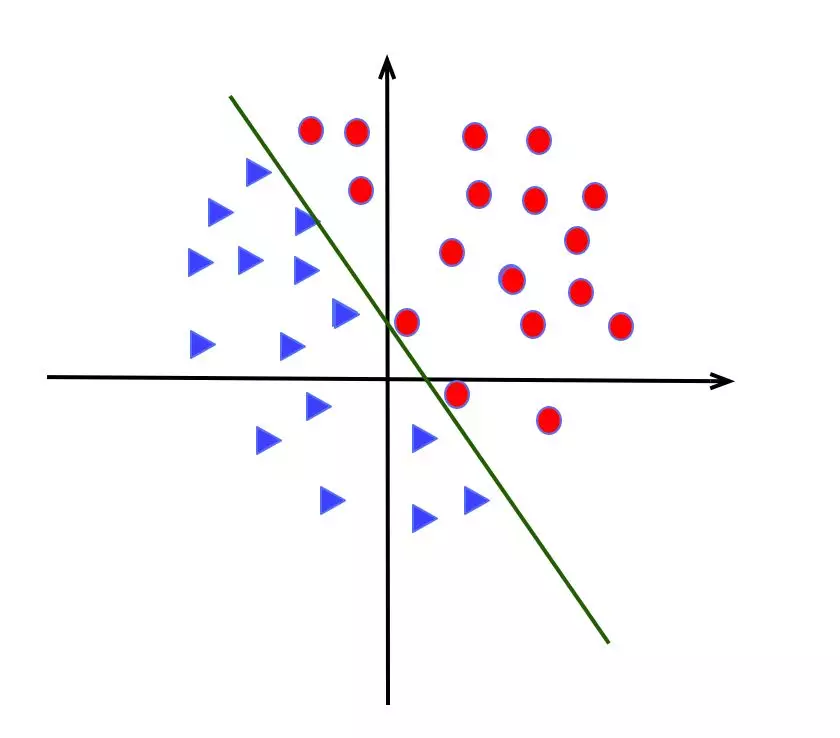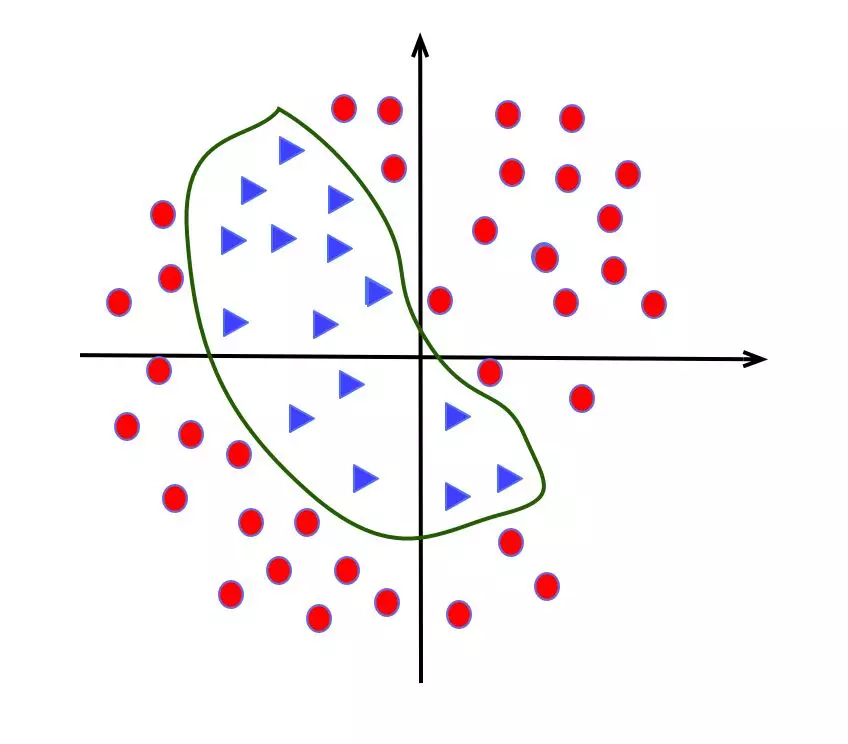### 二分类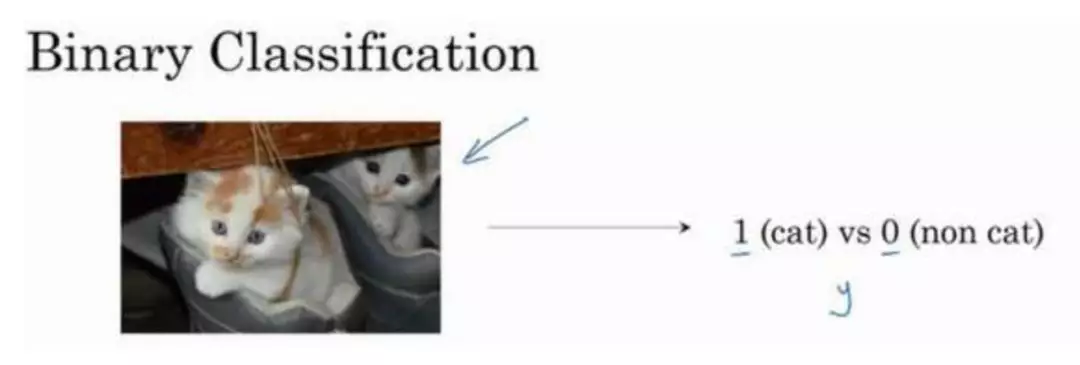### 逻辑回归模型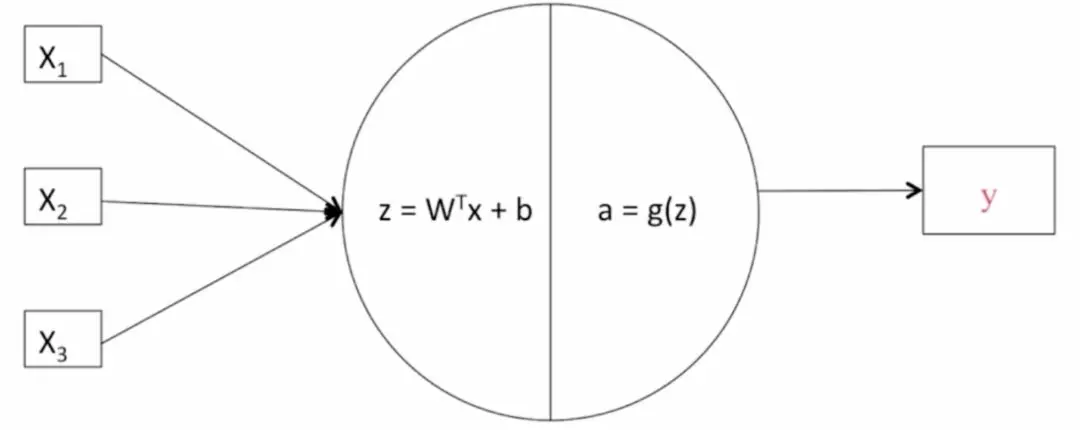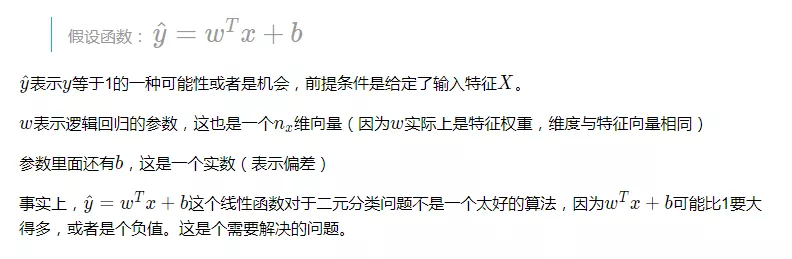sigmoid 函数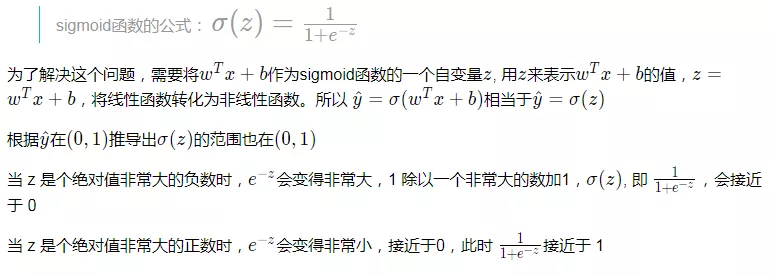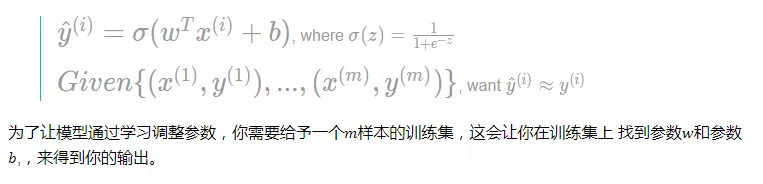Sigmoid 函数

tanh 函数

ReLU 函数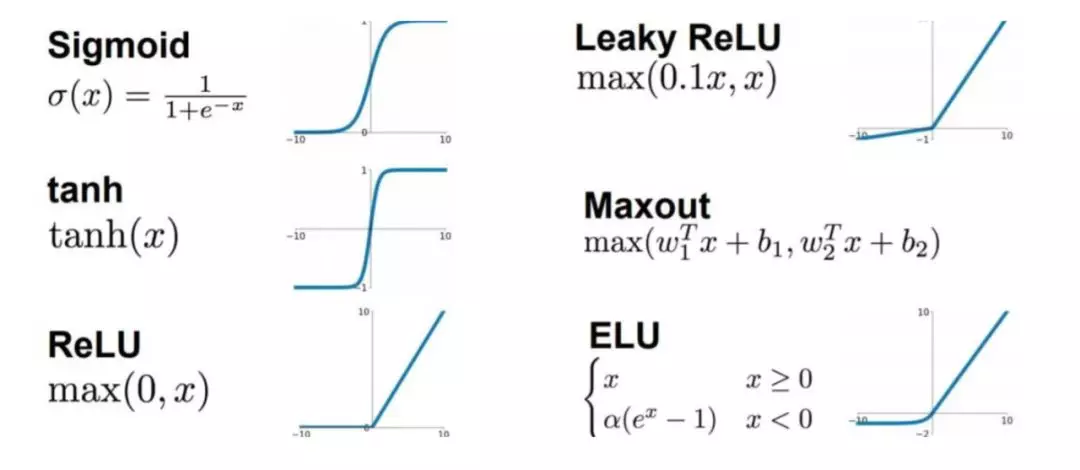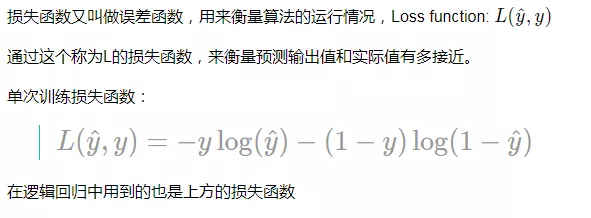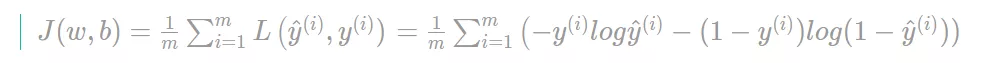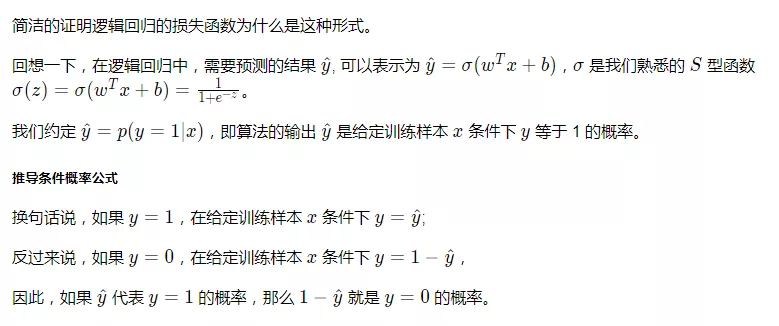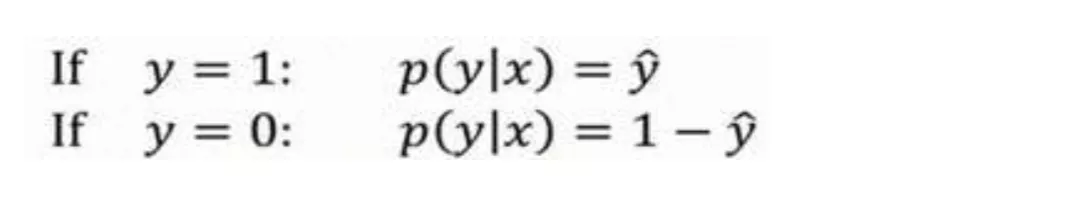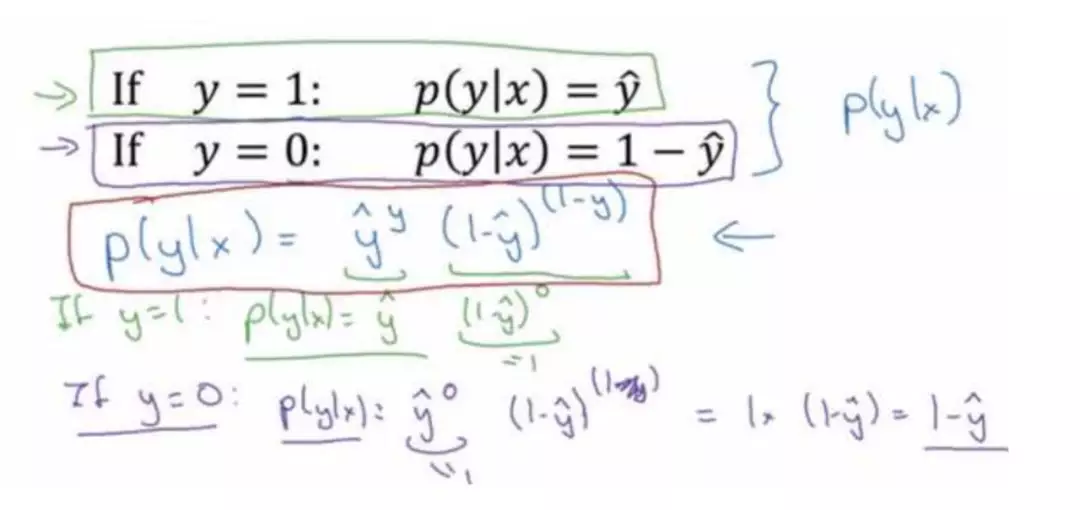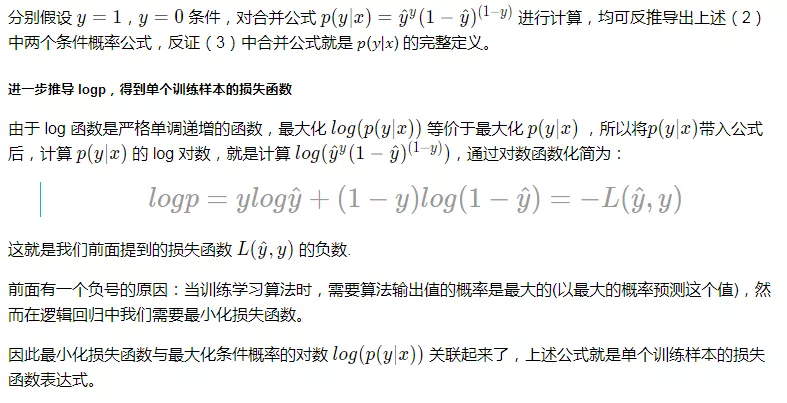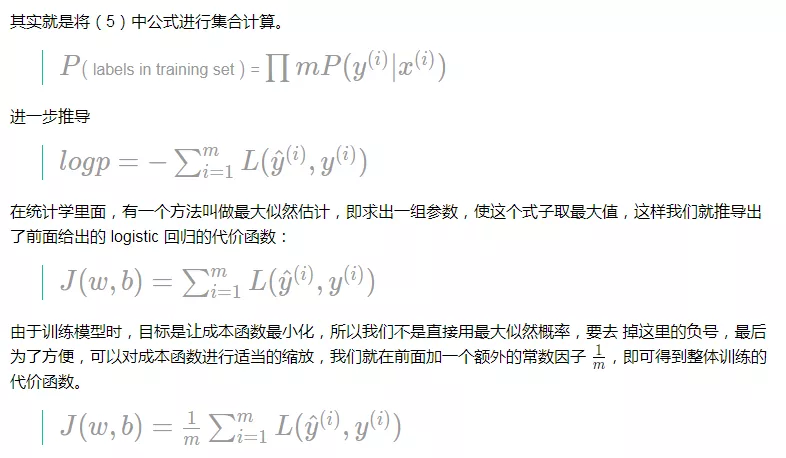### 计算图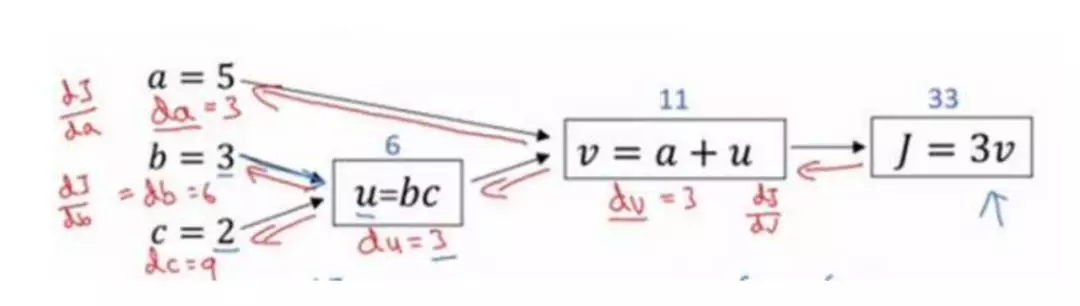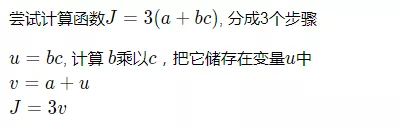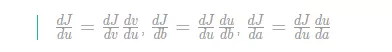### 梯度下降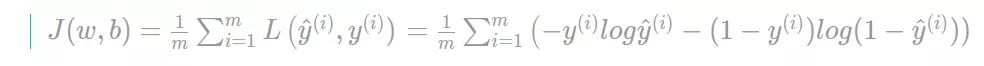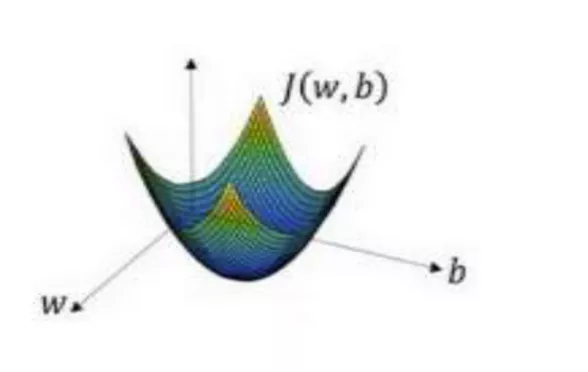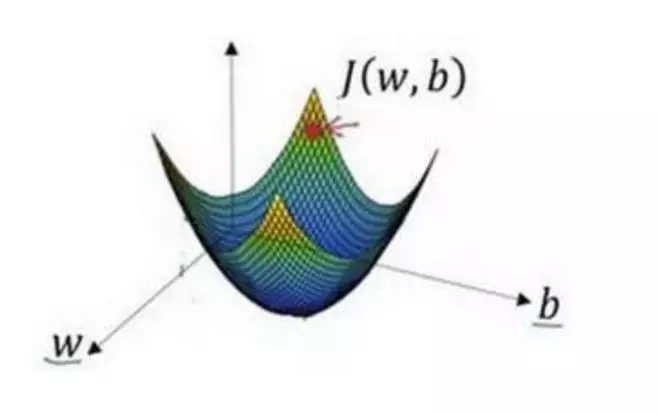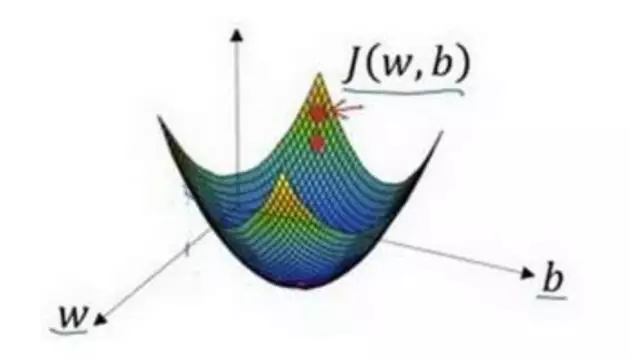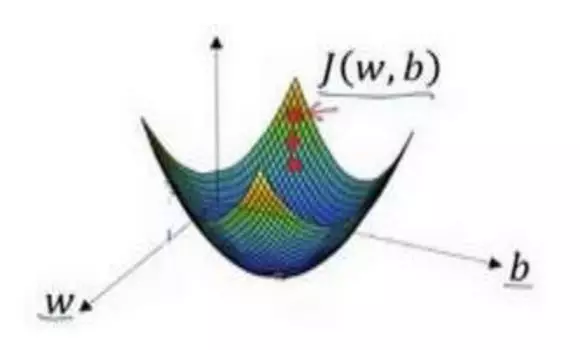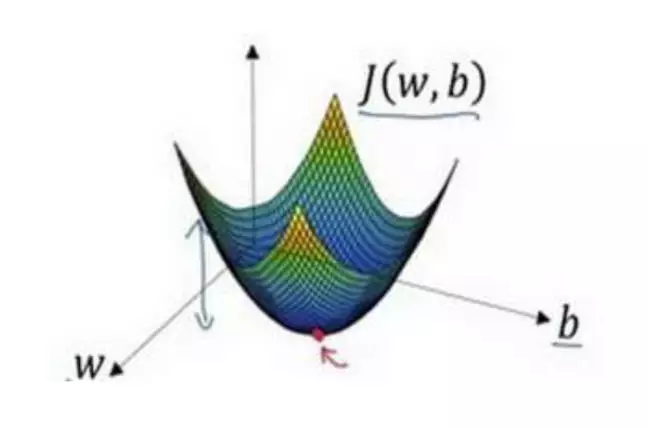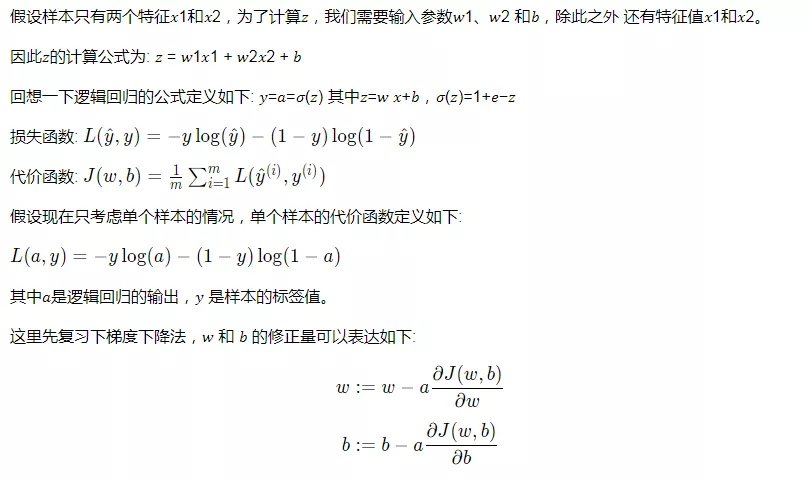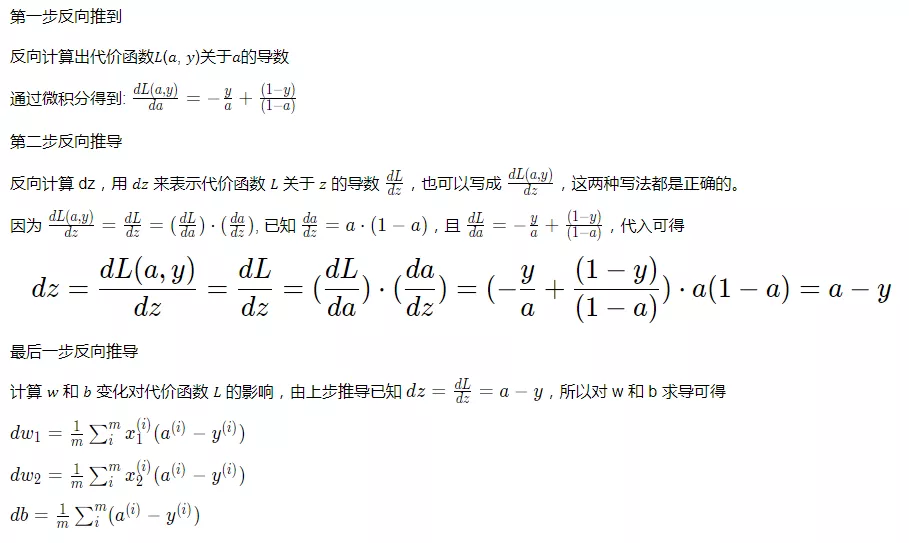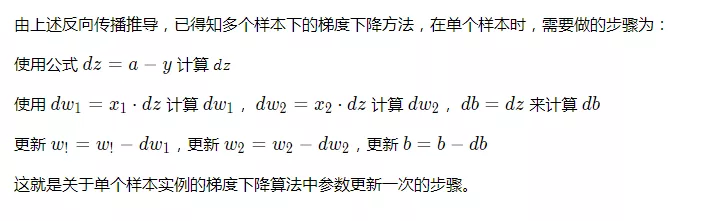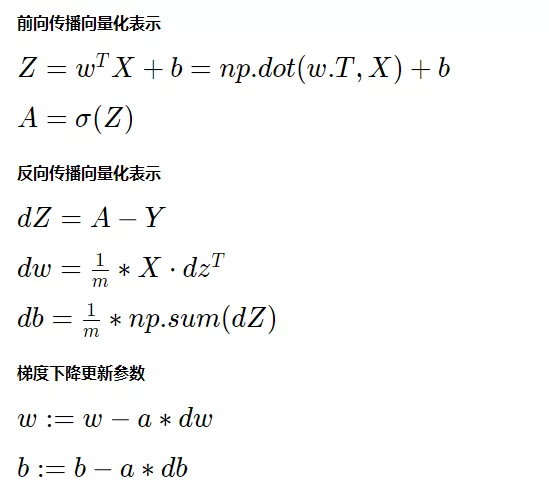1、引入外部包

2、加载数据集

3、定义计算图

4、初始化参数

5、一次迭代的前向传播：执行计算图，一层层计算，直到计算到输出层，得到预估值、准确率、损失函数

6、一次迭代的反向传播：对上次得到损失函数求导，使用优化函数（梯度下降算法）从右向左对神经网络的每一层计算调参，直到计算到第一个隐含层，得到优化更新的参数

7、重复（5）（6）为一个迭代，记录多个迭代的信息，训练完毕的模型的最终损失和准确率。

8、使用（7）中得到的训练过程数据，绘制训练集、测试集关于迭代次数与损失的关系曲线图以及迭代次数与准确率关系的曲线图，进行分析，以调整超参数。

## 第二章逻辑回归（Logistic Regression）实现猫咪识别器

import numpy as npimport matplotlib.pyplot as pltimport h5pyfrom PIL import Imagefrom loadH5File import load_data %matplotlib inline

train_set_x, train_set_y, test_set_x, test_set_y, classes = load_data()

train_x (209, 64, 64, 3) test_x (50, 64, 64, 3)train_y (1, 209) test_y (1, 50)

### 查阅训练集中的一张图片

index = 7plt.imshow(train_set_x[index])print ("y = " + str(train_set_y[:,index]) + ", it's a '" + classes[np.squeeze(train_set_y[:,index])].decode("utf-8") +  "' picture.")

y = , it's a 'cat' picture.index = 10plt.imshow(train_set_x[index])print ("y = " + str(train_set_y[:,index]) + ", it's a '" + classes[np.squeeze(train_set_y[:,index])].decode("utf-8") +  "' picture.")

y = , it's a 'non-cat' picture.png### 猫咪图片数据处理过程示意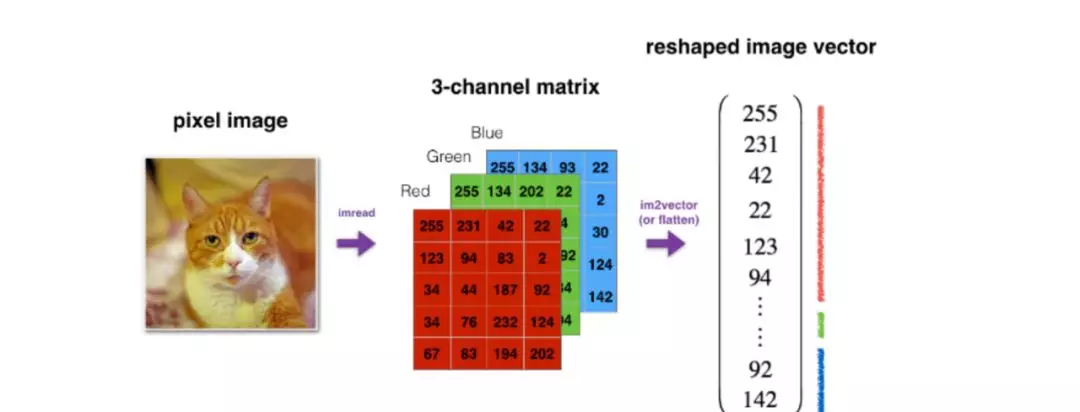reshape 目的：实现矩阵乘法计算

• x 维度切分为 (num_px * num_px * 3, m) 的形式。
• y 维度切分为 (1, m) 的形式。

num_px = train_set_x.shapem_train = train_set_x.shapem_test = test_set_x.shape # 重塑输入数据train_x_flatten = train_set_x.reshape(train_set_x.shape, -1).Ttest_x_flatten = test_set_x.reshape(test_set_x.shape, -1).T print('train_x', train_x_flatten.shape, 'test_x', test_x_flatten.shape)  print('train_y', train_set_y.shape, 'test_y', test_set_y.shape)    # 标准化输入数据train_set_x = train_x_flatten/255test_set_x = test_x_flatten/255

train_x (12288, 209) test_x (12288, 50)train_y (1, 209) test_y (1, 50)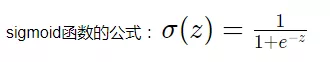def sigmoid(z):    '''    计算 z 的非线性输出值{1}    Arguments:    z -- 一个标量或者一个 numpy array{1}    Return:    s -- sigmoid(z)    '''    s = 1 / (1 + np.exp(-z))     return s

### 实现初始化参数函数def initialize_with_zeros(dim):    """    将 w 构造为一个 shape 为 (dim, 1) 的零值向量，将 b 设置为 0{1}    Argument:    dim -- 行向量 w 的大小{1}    Returns:    w -- 初始化后的行向量， shape 为 (dim, 1)    b -- 初始化后的标量 (表示偏差)    """     w = np.zeros(shape=(dim, 1))    b = 0     assert(w.shape == (dim, 1))    assert(isinstance(b, float) or isinstance(b, int))     return w, b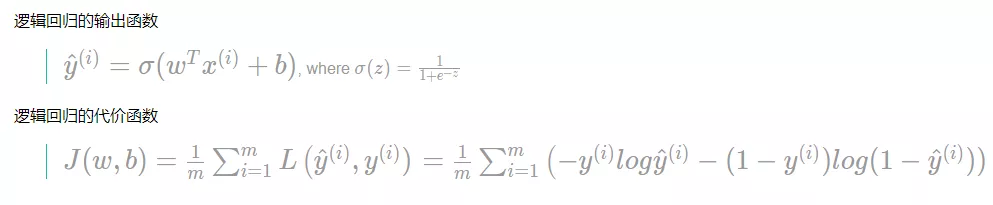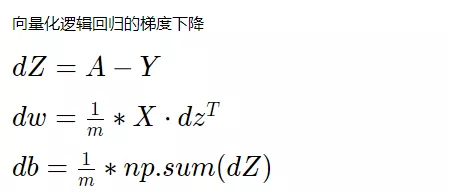def propagate(w, b, X, Y):    '''    实现前向传播和反向传播{1}    Arguments:    w -- 权重, 一个行向量 (num_px * num_px * 3, 1)    b -- 偏差, 一个标量    X -- 标准化后的 X 矩阵 (num_px * num_px * 3, number of examples){1}    Return:    cost -- 逻辑回归的成本函数（负值）    dw -- w 的梯度下降，shape 同 w     db -- b 的梯度下降，shape 同 b{1}    '''    m = X.shape     # 前向传播    A = sigmoid(np.dot(w.T, X) + b)    cost = -1 / m * np.sum(Y * np.log(A) + (1-Y) * np.log(1-A))     # 反向传播    dw = 1 / m * np.dot(X, (A-Y).T)    db = 1 / m * np.sum(A-Y)     assert(dw.shape == w.shape)    assert(db.dtype == float)    cost = np.squeeze(cost)    assert(cost.shape == ())     grads = {"dw": dw,             "db": db}     return grads, cost

### 实现调参函数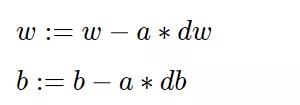def optimize(w, b, X, Y, num_iterations, learning_rate, print_cost = False):    '''    运行梯度下降算法，优化调整 w, b 参数     Arguments:    w -- 权重, 一个行向量 (num_px * num_px * 3, 1)    b -- 偏差, 一个标量    X -- 标准化后的 X 矩阵，shape 为 (num_px * num_px * 3, number of examples)    Y -- 一个由 0， 1 组成 (其中 0 表示 non-cat, 1 表示 cat) 的列向量, shape 为 (1, number of examples)    num_iterations -- 迭代次数 / 优化更新次数    learning_rate -- 梯度下降的学习率 / 更新步长    print_cost -- 是否打印损失，默认不打印     Returns:    params -- 一个包含了权重 w 和 偏差 b 的字典    grads -- 一个包含了权重 w 和 偏差 b 的梯度的字典    costs -- 一个保留了所有更新计算过的损失值的列表，用于绘制学习曲线    '''     costs = []     for i in range(num_iterations):        # 调用 propagate 函数计算 w, b 的梯度以及损失        grads, cost = propagate(w, b, X, Y)         dw = grads["dw"]        db = grads["db"]        # 更新 w, b，优化调整参数        w = w - learning_rate * dw        b = b - learning_rate * db        # 保存当次更新的损失        if i % 100 == 0:            costs.append(cost)        # 每训练 100 次，打印一次损失        if print_cost and i % 100 == 0:            print("Cost after iteration %i: %f" % (i, cost))     params = {"w": w,              "b": b}     grads = {"dw": dw,             "db": db}     return params, grads, costs